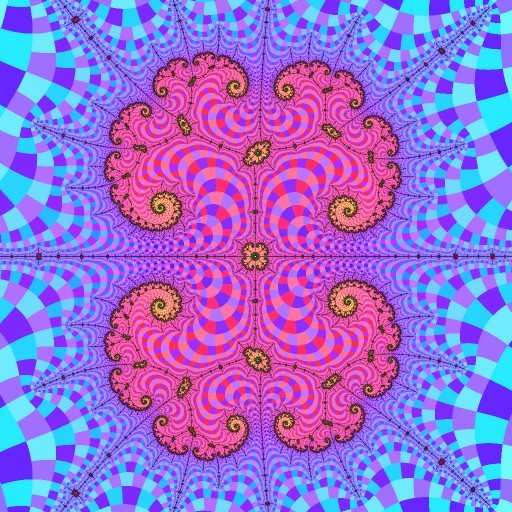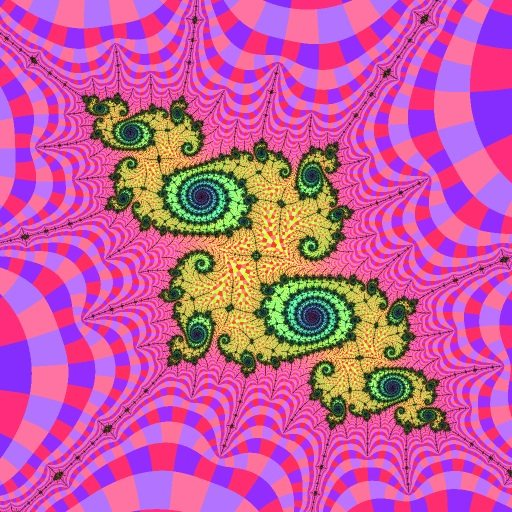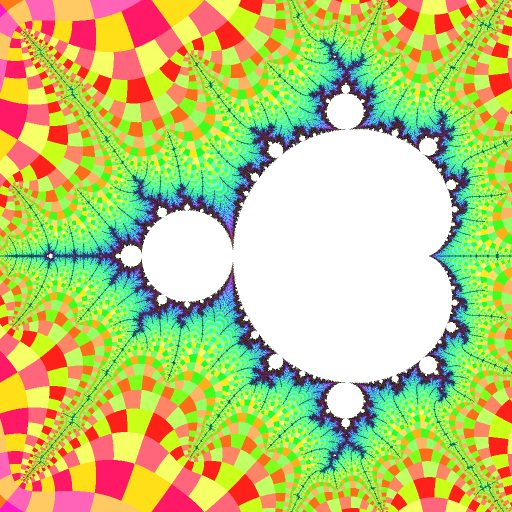# Embedded Julia Set

Robert P. Munafo, 2008 Feb 29

A structure comprised of filaments, resembling a Julia set in appearance, which has a higher delta Hausdorff dimension than filaments in the immediately surrounding region. They are sometimes also called Julia islands or virtual Julia Sets.

Embedded Julia sets are located "close" to mu-molecules. That is to say, for any given embeddded Julia set, there is a mu-molecule whose distance from the embedded Julia set is low compared to the mu-molecule's size. The continent is not counted in this definition. This mu-molecule is colloquially referred to as the influencing island mu-molecule. For example, R2F(1/2B1)S is the influencing island mu-molecule for the embedded Julia set in the first illustration below.

## Size

The size of an embedded Julia set is proportional to the size of its influencing mu-molecule, and inversely proportional to its distance from the influencing mu-molecule, or distance from other larger-size embedded Julia Sets (whichever distance is less).

## Shape

All Embedded Julia Sets are shaped like a Julia Set, and specifically, a Fatou dust (Cantor set). The shape of the Embedded Julia Set is very close to (but never exactly the same as) the Julia set whose Parameter whose position relative to the Continent corresponds to the embedded Julia set's position relative to the influencing island. The examples below illustrate this.

There are three canonical types of embedded Julia Set shapes, although most really fall on a continuum somewhere between these extremes:

Type C (C is for cusp) embedded Julia sets are those found in cusps. There are two subclasses, type C and type CC (discussed next). All type C's have large open regions with clearly visible nodes, and the filaments connecting the center through the nodes to the Fatou dust have an obvious binary-tree structure. Figures 1 and 2 below exemplify type C.

Type CC (CC is for cardioid cusp) embedded Julia sets are a special and very important subclass of type C. They are all shaped like the cauliflower Julia set. This Julia set has a single component and a totally connected interior, and the nodes are connected directly to one another without going through Fatou dust boundaries. Figure 1 below is a type CC.

Type F (F is for filament) embedded Julia sets are found near a filament that is not in a cusp. They are shaped like filaments themselves, and the nodes are difficult to distinguish because there are no open spaces with nodes in the middle. However, the nodes can still be seen because they are places where the filaments become "hairier" (areas of the embedded Julia set that have a locally higher Hausdorff dimension). Figure 3 below is a type F.

## Major Features

Embedded Julia Sets have three distinct notable components, and only one of these is the part that actually resembles the Fatou Dust.

The Fatou Dust portion defines the overall boundary and shape of the Embedded Julia Set. It consists of many disconnected pieces, all seemingly identical to each other except for minor distortions, rotation and size differences.

The second component is a set of Filaments whose shape is very similar to the filaments adorning the influencing island, which in turn resemble the filament of which the influencing island is a part.

The third and perhaps most interesting part of the Enbedded Julia Sets are the miniature self-squared images (which I call paramecia or nodes) that appear around all islands within the filaments. There is always one at the center of the Embedded Julia Set, whose shape has 4-way rotational symmetry (demonstrating bifurcation), but there are also many others all with non-symmetrical shapes, and all different from each other.

## Examples

The largest embedded Julia sets in the Mandelbrot Set are located in the vicinity of R2F(1/2B1)S, which is the largest island. Here are some representative views of embedded Julia sets in that region:-1.74876455 + 0 i @ 2.4e-5 ~.. -, -( , .- '"., T ] [ . ./ __*- `., \ e,c____.. [ c_'_-.' .ac` `-_ -_)\$""^/""*Ye:\_)/(@"\$d\e,~ ..-' .,'-.@m- T` -@L[]-Ymbc `r-~"Y)_.-'` `-.dF"@--._", '' ] '^' [ '"FL%e, . `--.,_,'.FF`, _%Y)\. ,'_ .__Ym,`'*'\$m,^ ^'_@;"Y"^^ ^`-.d@\$)^'' ' '-vm'-FL_,---'' '"(b\/^ , -^^' ',:\$e_ ^^^^:C.[ .ab(,- [ ._a___ T`@^-,- --'*/e'**/F- -) ,dC:d`a(/`L '`'^""F"' .__[,, -^Y(**`^ -.--:----:-------------,----]T@@---.----------.--c-:-m--,.,- _ .a,,-.. -'"F" ,e:a__-_ -__L\F^L%Ye. .L -aF%\$^")mr- ____vm"/ '@@d"- [ -**-"; T:b`--- ._\$F/L `' _`., :"~YF- _"bca)_, ._.-@\$(-__ .."d-(@"---.,_ _,,--^^^mC[d^`'^-:e-^^` ~^^ '^^-am~ __`v@% '^ ` _."@L.m,_'*^` . ] _. %`'./F""` ` ` '^^"/m T, adme'-dmd- aL_dT\$``-_, _,^ .Ymv.^-\_^%)[]'YmL,v`:r/F"/ '~_, _-~ /`""FF-"""^ -c `/^^^^', ' '`\/, __-` . ] '[ ' '\ `-_, .'" a` % '` ^-. -1.74876455 +0.00000001 i @ +0.00002441, Nmax: 1000
Figure 1. This is near R2F(1/2B1)S.C(0), the main cusp or the elephant valley of R2F(1/2B1)S. Inside this Embedded Julia Set are many smaller, similar features with various distorted shapes; see the paramecia heading for pictures.-1.768620774 + 0.002428273 i @ 9e-6 _[ -,,^ ,, '^ ."` `-,. ] ]` .-` ^^-, ', . " : :a' `-._ea_,..] .,_,, ., :` .~ -^Y@@F@bdm)b@@Ce.~ d . ./` d.@b(@@@@m-"^Y@[-,...a`,. ].._/` ^^`^`.`**@@F*\_/"@/"e)dmm@@me-\dd@@;__, ___%d@*`,._m"d@@@dbLd";`d@@@@@*^` _ -_@bd@"d@F@@@@@@FTLa__,'@@@^b,: _.--^` _.,-"YY"C/cFb%@@@@@\\$@` 'F`:--]@@m^'` _.__--^'^ `'`'"*Y(vY*@vF@"^' ' da_d.dC_-- ` --~ ^`v.addb/,--:b-- -*@@FY~`_, _,-` ,.a_ -^^,d@-Y*L`. \eem\$mebad(mLa_/, , __--'^ ' ___."d@Fe,- .^ '/@FCb@@@@-@%"FFmaa_,-'` --^^^ .:,Ybamde^^^.(@\Y\$@@@@@/@m//Y@@F`` ___:a@@@@bmc^.-*Yd\$@@@@@d@* `aYY@,--^` -:@@@F":@bdmm%bmb"/%%C`-"-d*@*;,.- . __ .,'` .`':-'"*``"``~\$C*` ,(d@@@@@@eer-^'^` .a-^ - a ` '_FYLbeC^FY@@@Y^\$)^ _ -,.-` ./ ./` :Y@@"YFY^YCa@@@b%` a,^~ .` ' .^' [ '`'""'F"`-_, .,..-` ` _` ` ', ' '-._ ._'" ' ', ' e,,-` ]` -1.768620774 +0.002428273 i @ +0.000009000, Nmax: 1000
Figure 2. This is in R2F(1/2B1)S.C(1/2)-, the "seahorse valley" region of R2F(1/2B1)S.-1.7576852691 + 0.0176750996 i @ 3.8e-7 -, .` '", r` L / /` ., . '\_/ :` :` '-._-.-:@[' :" ., '_.- . '-m@F@@m/@mL-/ca~. [ ,`m@;.,-^` e__e.._--'- `^``:Y*b@bF`',\'(db@7"` _. ^ ' _- '_/"\$F7@dbaam@F""\^^^` . _,-~^ ` ' ~*` ^Y`"T@/-_._,- ___-~` ..-` -- d\$\$@bd.- , __"-~^ _,-`` ___/Y@@%~;-`\( .,-' ^ _:,.(@@:)@YF`^` __._***Fd@@@)a_,---^` ' -'aadFF@LCC"^ ., ...- '^"(^^'_*@Cee^'` _/-` ._,-` - -"@@@*\^_- ,-` .__--^ ---^`'@@a__e-amc.- . . a_,^^ m_ab@FT*@@b@@)L-,-`. .---^^^^'^' ,__-` ` ^'`,/@@@L,-:`-F@7Y@L)@dad`- ,~` __-^YC\' ', -'^'-:@//^^^-, -^ -^*,` ` c '.^`, [ '[ 'c :` ` -\ -/. '\ .` ` -1.7576852691 +0.0176750996 i @ +0.0000003814, Nmax: 1000
Figure 3 This is near R2F(1/2B1)SF(1/3B*), the point on R2F(1/2B1)S that corresponds to R2F(1/3B*) on the main Mandelbrot Set.-1.76733 +0.00002 i @ 0.05 ", 3 _ ./ '", . .` :c( `., '.\r-,-` . -~ ``\ -, -., ^-"@bbF'_,~ ..- ~_- '\, `-,.mL@F@La\ . a` ''-, `_ \ v -.\$F ^@` /,: /_,._ '-_ _ `\bade\b)@*---"""(*b.mm@b~_ . '^. 'c -, \TC@^""` `"`_dF`_.--' ~_a , :. , _`m@""` 'Ye_b\, '. ., --]@)_cmdeL.'-@F` \FY___- `e `.,/-,"@^*""""\e:F 2 :%*L, , ,'-. - - -m)\$@^ 'Y :F" .-(':ddddaddm@d@^^` R2F(1/2B1)Sa -m^------ 1 ~ ''/''/',^d@Y(b, .a Y_r _" _/`\;"\$@-_,...a/T, '(L^^ .-` -` .,:)Y*F@bFF\-\$bc .C7---- .."^`` :^ ` ^a@@L, ,r(@r- ._-` :c .-`-^:@`.,, ._-'@;-`--__ .,^ ' ^a@@@FTbv__, ___a_m"bmd@,- ' .~ _-` `/'"`-~@C 'b''(`' '*"'` \$", :/` __--@bmcmbY)- ~ '\ a/` .~ -` '.;C@@e-`-, '~, _-` ' .m/@\`^^ ' '., ` ..' `~ . 'c/ .` " '\ -1.76733 +0.00002 i @ +0.05000, Nmax: 1000 R2F(1/2B1)S
Key map: picture of R2F(1/2B1)S showing locations of figures 1, 2 and 3. Locations of figures 1 and 2 are to the left of "1" and "2" in this illustration; figure 3 is below the point marked "3".

## Classes of Embedded Julia Sets

Embedded Julia Sets can be roughly grouped into classes based on various properties :

Where they are in relation to their influencing island: See the discussion above under the shape heading.

Their symmetry: Embedded Julia Sets are also classified by their symmetry. A 2-fold embedded Julia set has 2-fold rotational symmetry. See also n-fold embedded Julia set.

Their order: Beyond Embedded Julia Sets lie second-order embedded Julia sets. See that entry for more details.

## Why are They There?

The embedded Julia sets exist because of a combination of three different properties of the Mandelbrot set in general:

1. tuning: the phenomenon that causes small Julia sets to appear within other Julia sets when the parameter is in or near any mu-atom other than a secondary continental mu-atom or the continent seed. This is a well-known and frequently discussed phenomenon; for illustrations see for example [Milnor1999Loc] pages 36,37 and [Milnor1999Per] pages 40,41.

2. For any C, the region of the Mandelbrot set near C is locally similar to the Julia set with parameter value C near the point Z=C, usually with a change in size and rotation. This has been known since the early days. Most Mandelbrot programs that allow you to switch between "Julia mode" and "Mandelbrot mode" make it easy to see the similarity.

3. "renormalization" (period scaling): This is closely related to "tuning", and is is discussed for example in [Milnor1999Per] pages 36-40 and [Riedl2000] pages 48-50. It is necessary for the existence of second-order embedded Julia sets.

In other words, because Julia Sets appear inside other Julia Sets (see for example figures 6, 7 and 8 on page 9 of [Milnor1999Per]), and because every part of the Mandelbrot Set locally resembles a Julia Set of the same parameter value, we see Julia Sets within the Mandelbrot Set.

## Further Speculation on Their Origin

To understand why Julia sets appear inside other Julia sets requires a much deeper understanding of the orbital dynamics. In the papers I have seen, the math and the language of the exposition is too hard for me to grasp, or for others I have spoken with to explain.

However, a lot of what is going on can be observed. One can observe a real Julia set, and compare it to an embedded Julia set with the same shape. Of course it is a Fatou dust, a Cantor set, an uncountable number of points.

In the Fatou set, consider the center, which is the origin, and a number of points that are pre-images of the origin. Two pre-images are "order one", if they are iterated once using the formula z2+c, the iterate is 0. (Because it is a Julia set, c is the same for all points). There are four pre-images of order two, points z for which (z2+c)2+c = 0. And eight pre-images of order three, etc.

If you look at the embedded Julia set, you find an island (baby Mandelbrot) at the center. Let's look at the one centered at -1.76886792 +0.00392602 i (set your view to a square 0.000025 units on a side to see the entire embedded Julia set). The island has period 44. The islands corresponding to the 1st-order pre-images are at -1.7688720239 +0.0039323033 i and at -1.7688635553 +0.0039192692 i, and have period 47. There are four period-50 islands, at -1.7688663431 + 0.0039289815 i, and -1.7688696374 + 0.0039230778 i, and -1.76886102889 + 0.00391618279 i , and -1.76887415336 + 0.00393486813 i . (For all of these islands, set the size of your view to around 10-8 and you'll see the island). There are eight period-53 islands, and so on.

Now go back to the Fatou dust. Each point in the Fatou dust is the limit of a sequence of pre-images. I don't know how you select the pre-images to make it fit a proof, but it's clear from the pictures that the pre-images get denser and denser as you approach the actual Fatou dust points.

So far, all this is just observation. Why does it actually take place?

In the example, the islands' periods increase by three each time you go to the next higher set of pre-images (44{->}47{->}50 and so on). I believe that is because of the period-3 orbital dynamics imposed by the large island that we are near (the period-3 island at -1.75+0i). The little islands of period-44 exists because, in a small region, you can iterate 44 times and end up back where you started.

It is my belief that 3 of those 44 iterations are equivalent to a Julia iteration z2+K for some constant K related to our location with respect to the period-3 island. It is like a Julia iteration with some rotation and scaling, and a fairly small non-linear distortion. For this reason, there are two places (the pre-images of the origin) where you can iterate 47 times and end up back where you started — because three extra iterations get cancelled out by the application of this z2+K=0. Similarly, there are 4 places with period 50=44+6, and so on. It would follow from this that the baby Mandelbrots converge on a limit set similar in appearance to the Julia set for constant K.

revisions: 20080229 oldest on record; 20100925 add example of 'influencing' term.

From the Mandelbrot Set Glossary and Encyclopedia, by Robert Munafo, (c) 1987-2020.     Mu-ency index

This page was written in the "embarrassingly readable" markup language RHTF, and was last updated on 2020 Jan 15.s.11